# Pandas DataFrame Plot scatter graph

Pandas plot

Pandas.DataFrame.plot to generate scatter graph using data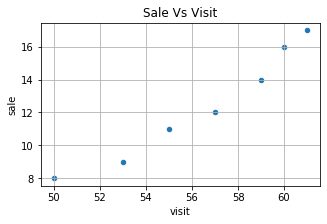``````import pandas as pd
my_dict={
'visit':[50,53,55,57,59,60,61],
'sale':[8,9,11,12,14,16,17]
}
df =pd.DataFrame(data=my_dict)
df.plot.scatter(title='Sale Vs Visit',x='visit',y='sale')``````

## scatter chart with options

There are several options we can add to above scatter diagram.

## title :

title='sale Vs Visit' String used as Title of the graph.

## figsize :Size of the graph , it is a tuple saying width and height in inches, figsize=(6,3). Here width is 6 inches and height is 3 inches.

``df.plot.scatter(figsize=(6,3),x='visit',y='sale')``

## fontsize

fontsize=20 , we can set the font size used labels in x and y axis.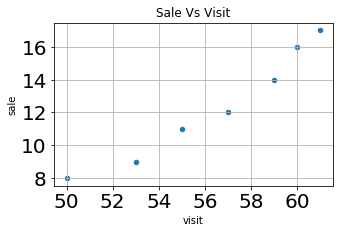``df.plot.scatter(x='visit',y='sale',fontsize=20)``

## color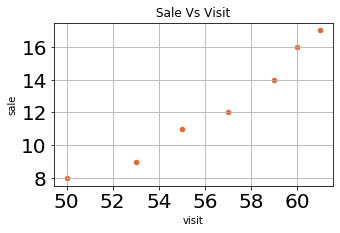We can use the option colors to give different colors to points . We can use one tuple to define the colours. Here it is color=[(.9,.4,.2)], this is in R G B format where each value varies from 0 to 1.
``df.plot.scatter(title='Sale',x='visit',y='sale',color=[(.9,.4,.2)])``

## grid

We will show grid ( grid=True ) or not ( grid=False)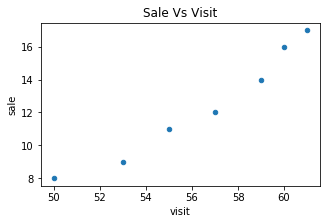``df.plot.scatter(title='grid=True',x='visit',y='sale',grid=True)``

## logx logy loglog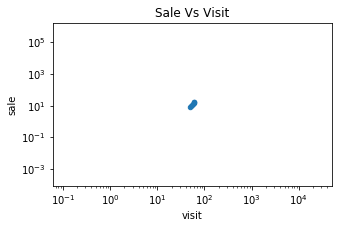We can specify log scaling or symlog scaling for x ( logx=True ) or log scaling for y ( logy=True ), we can specify both the axis by loglog ( loglog=True)

``df.plot.scatter(loglog=True,x='visit',y='sale')``

## rot

Rotation of ticks ( check the label at x axis )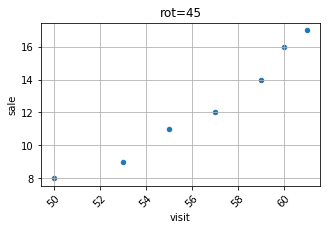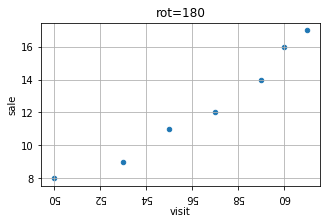``df.plot.scatter(title='rot=180',x='visit',y='sale')``

Subscribe to our YouTube Channel here

## Subscribe

* indicates required
Subscribe to plus2netplus2net.com